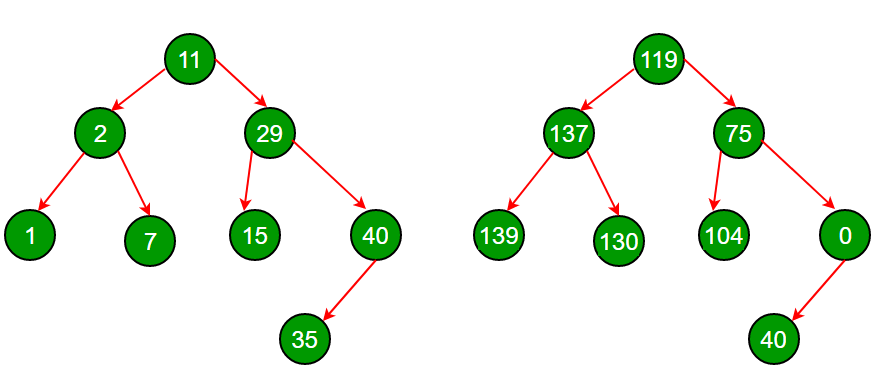Related Articles
Transform a BST to greater sum tree
• Difficulty Level : Medium
• Last Updated : 19 Feb, 2021

Given a BST, transform it into a greater sum tree where each node contains sum of all nodes greater than that node.We strongly recommend to minimize the browser and try this yourself first.
Method 1 (Naïve):
This method doesn’t require the tree to be a BST. Following are the steps.
1. Traverse node by node(Inorder, preorder, etc.)
2. For each node find all the nodes greater than that of the current node, sum the values. Store all these sums.
3. Replace each node value with their corresponding sum by traversing in the same order as in Step 1.
This takes O(n^2) Time Complexity.

Method 2 (Using only one traversal)
By leveraging the fact that the tree is a BST, we can find an O(n) solution. The idea is to traverse BST in reverse inorder. Reverse inorder traversal of a BST gives us keys in decreasing order. Before visiting a node, we visit all greater nodes of that node. While traversing we keep track of the sum of keys which is the sum of all the keys greater than the key of the current node.

## C

 `// C++ program to transform a BST to sum tree``#include``using` `namespace` `std;` `// A BST node``struct` `Node``{``    ``int` `data;``    ``struct` `Node *left, *right;``};` `// A utility function to create a new Binary Tree Node``struct` `Node *newNode(``int` `item)``{``    ``struct` `Node *temp =  ``new` `Node;``    ``temp->data = item;``    ``temp->left = temp->right = NULL;``    ``return` `temp;``}` `// Recursive function to transform a BST to sum tree.``// This function traverses the tree in reverse inorder so``// that we have visited all greater key nodes of the currently``// visited node``void` `transformTreeUtil(``struct` `Node *root, ``int` `*sum)``{``   ``// Base case``   ``if` `(root == NULL)  ``return``;` `   ``// Recur for right subtree``   ``transformTreeUtil(root->right, sum);` `   ``// Update sum``   ``*sum = *sum + root->data;` `   ``// Store old sum in current node``   ``root->data = *sum - root->data;` `   ``// Recur for left subtree``   ``transformTreeUtil(root->left, sum);``}` `// A wrapper over transformTreeUtil()``void` `transformTree(``struct` `Node *root)``{``    ``int` `sum = 0; ``// Initialize sum``    ``transformTreeUtil(root, &sum);``}` `// A utility function to print indorder traversal of a``// binary tree``void` `printInorder(``struct` `Node *root)``{``    ``if` `(root == NULL) ``return``;` `    ``printInorder(root->left);``    ``cout << root->data << ``" "``;``    ``printInorder(root->right);``}` `// Driver Program to test above functions``int` `main()``{``    ``struct` `Node *root = newNode(11);``    ``root->left = newNode(2);``    ``root->right = newNode(29);``    ``root->left->left = newNode(1);``    ``root->left->right = newNode(7);``    ``root->right->left = newNode(15);``    ``root->right->right = newNode(40);``    ``root->right->right->left = newNode(35);` `    ``cout << ``"Inorder Traversal of given tree\n"``;``    ``printInorder(root);` `    ``transformTree(root);` `    ``cout << ``"\n\nInorder Traversal of transformed tree\n"``;``    ``printInorder(root);` `    ``return` `0;``}`

## Java

 `// Java program to transform a BST to sum tree``import` `java.io.*;``class` `Node``{``  ``int` `data;``  ``Node left, right;` `  ``// A utility function to create a new Binary Tree Node``  ``Node(``int` `item)``  ``{``    ``data = item;``    ``left = right = ``null``;``  ``}``}` `class` `GFG``{` `  ``static` `int` `sum = ``0``;``  ``static` `Node Root;` `  ``// Recursive function to transform a BST to sum tree.``  ``// This function traverses the tree in reverse inorder so``  ``// that we have visited all greater key nodes of the currently``  ``// visited node``  ``static` `void` `transformTreeUtil(Node root)``  ``{` `    ``// Base case``    ``if` `(root == ``null``) ``      ``return``;` `    ``// Recur for right subtree``    ``transformTreeUtil(root.right);` `    ``// Update sum``    ``sum = sum + root.data;` `    ``// Store old sum in current node``    ``root.data = sum - root.data;` `    ``// Recur for left subtree``    ``transformTreeUtil(root.left);``  ``}` `  ``// A wrapper over transformTreeUtil()``  ``static` `void` `transformTree(Node root)``  ``{` `    ``transformTreeUtil(root);``  ``}` `  ``// A utility function to print indorder traversal of a``  ``// binary tree``  ``static` `void` `printInorder(Node root)``  ``{``    ``if` `(root == ``null``)``      ``return``;``    ``printInorder(root.left);``    ``System.out.print(root.data + ``" "``);``    ``printInorder(root.right);``  ``}` `  ``// Driver Program to test above functions``  ``public` `static` `void` `main (String[] args) {` `    ``GFG.Root = ``new` `Node(``11``);``    ``GFG.Root.left = ``new` `Node(``2``);``    ``GFG.Root.right = ``new` `Node(``29``);``    ``GFG.Root.left.left = ``new` `Node(``1``);``    ``GFG.Root.left.right = ``new` `Node(``7``);``    ``GFG.Root.right.left = ``new` `Node(``15``);``    ``GFG.Root.right.right = ``new` `Node(``40``);``    ``GFG.Root.right.right.left = ``new` `Node(``35``);` `    ``System.out.println(``"Inorder Traversal of given tree"``);``    ``printInorder(Root);` `    ``transformTree(Root);``    ``System.out.println(``"\n\nInorder Traversal of transformed tree"``);``    ``printInorder(Root);``  ``}``}` `// This code is contributed by avanitrachhadiya2155`

## Python3

 `# Python3 program to transform a BST to sum tree` `class` `Node:``    ``def` `__init__(``self``, x):``        ``self``.data ``=` `x``        ``self``.left ``=` `None``        ``self``.right ``=` `None` `# Recursive function to transform a BST to sum tree.``# This function traverses the tree in reverse inorder so``# that we have visited all greater key nodes of the currently``# visited node``def` `transformTreeUtil(root):``  ` `   ``# Base case``   ``if` `(root ``=``=` `None``):``        ``return` `   ``# Recur for right subtree``   ``transformTreeUtil(root.right)` `   ``# Update sum``   ``global` `sum``   ``sum` `=` `sum` `+` `root.data` `   ``# Store old sum in current node``   ``root.data ``=` `sum` `-` `root.data` `   ``# Recur for left subtree``   ``transformTreeUtil(root.left)` `# A wrapper over transformTreeUtil()``def` `transformTree(root):` `    ``# sum = 0 #Initialize sum``    ``transformTreeUtil(root)` `# A utility function to prindorder traversal of a``# binary tree``def` `printInorder(root):``    ``if` `(root ``=``=` `None``):``        ``return` `    ``printInorder(root.left)``    ``print``(root.data, end ``=` `" "``)``    ``printInorder(root.right)` `# Driver Program to test above functions``if` `__name__ ``=``=` `'__main__'``:` `    ``sum``=``0``    ``root ``=` `Node(``11``)``    ``root.left ``=` `Node(``2``)``    ``root.right ``=` `Node(``29``)``    ``root.left.left ``=` `Node(``1``)``    ``root.left.right ``=` `Node(``7``)``    ``root.right.left ``=` `Node(``15``)``    ``root.right.right ``=` `Node(``40``)``    ``root.right.right.left ``=` `Node(``35``)` `    ``print``(``"Inorder Traversal of given tree"``)``    ``printInorder(root)` `    ``transformTree(root)` `    ``print``(``"\nInorder Traversal of transformed tree"``)``    ``printInorder(root)` `    ``# This code is contributed by mohit kumar 29`

Output:

```Inorder Traversal of given tree
1 2 7 11 15 29 35 40

Inorder Traversal of transformed tree
139 137 130 119 104 75 40 0```

The time complexity of this method is O(n) as it does a simple traversal of the tree.
https://youtu.be/hx8IADDBqb0?list=PLqM7alHXFySHCXD7r1J0ky9Zg_GBB1dbk

This article is contributed by Bhavana. Please write comments if you find anything incorrect, or you want to share more information about the topic discussed above

Attention reader! Don’t stop learning now. Get hold of all the important DSA concepts with the DSA Self Paced Course at a student-friendly price and become industry ready.

My Personal Notes arrow_drop_up